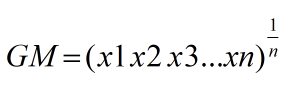Request a Tool

Geometric Mean Calculator

Geometric Mean Calculator is use to calculate the geometric mean of input data set.

Geometric Mean
0

Formula• GM = Geometric Mean
• x1x2...xn = Value of data set that multiplying
• n = Number of values

Defination / Uses

The geometric mean is a mean or average in mathematics that uses the product of two integers to illustrate the centre tendency or usual value of a set of numbers.

The geometric mean, also known as the geometric average, is a specialised average defined as the n-th root of the product of n numbers of the same sign. In an arithmetic mean, we sum the numbers and then divide by their number, whereas in a geometric mean, we calculate the product of the numbers and then take the n-th root. The geometric mean is the answer when there are multiple elements that contribute to a product and you wish to determine the "average" of the factors. It's helpful in a variety of circumstances where a growth rate is important.